Vibrations and Waves - Lesson 0 - Vibrations

# Properties of Periodic MotionA vibrating object is wiggling about a fixed position. Like the mass on a spring in the animation at the right, a vibrating object is moving over the same path over the course of time. Its motion repeats itself over and over again. If it were not for damping, the vibrations would endure forever (or at least until someone catches the mass and brings it to rest). The mass on the spring not only repeats the same motion, it does so in a regular fashion. The time it takes to complete one back and forth cycle is always the same amount of time. If it takes the mass 3.2 seconds for the mass to complete the first back and forth cycle, then it will take 3.2 seconds to complete the seventh back and forth cycle. It's like clockwork. It's so predictable that you could set your watch by it. In Physics, a motion that is regular and repeating is referred to as a periodic motion. Most objects that vibrate do so in a regular and repeated fashion; their vibrations are periodic. (Special thanks to Oleg Alexandrov for the animation of the mass on a spring. It is a public domain acquired from WikiMedia Commons. )

### The Sinusoidal Nature of a Vibration

Suppose that a motion detector was placed below a vibrating mass on a spring in order to detect the changes in the mass's position over the course of time. And suppose that the data from the motion detector could represent the motion of the mass by a position vs. time plot. The graphic below depicts such a graph. For discussion sake, several points have been labeled on the graph to assist in the follow-up discussion.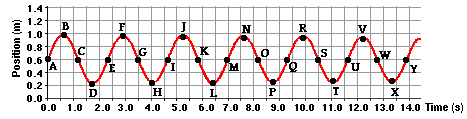Before reading on, take a moment to reflect on the type of information that is conveyed by the graph. And take a moment to reflect about what quantities on the graph might be important in understanding the mathematical description of a mass on a spring. If you've taken time to ponder these questions, the following discussion will likely be more meaningful.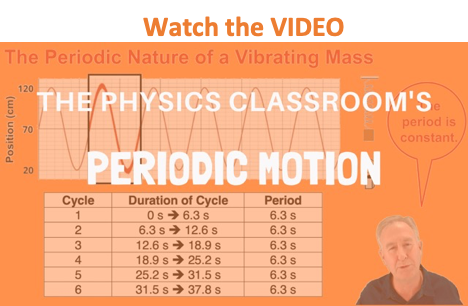One obvious characteristic of the graph has to do with its shape. Many students recognize the shape of this graph from experiences in Mathematics class. The graph has the shape of a sine wave. If y = sine(x) is plotted on a graphing calculator, a graph with this same shape would be created. The vertical axis of the above graph represents the position of the mass relative to the motion detector. A position of about 0.60 m cm above the detector represents the resting position of the mass. So the mass is vibrating back and forth about this fixed resting position over the course of time. There is something sinusoidal about the vibration of a mass on a spring. And the same can be said of a pendulum vibrating about a fixed position or of a guitar string or of the air inside of a wind instrument. The position of the mass is a function of the sine of the time.

A second obvious characteristic of the graph may be its periodic nature. The motion repeats itself in a regular fashion. Time is being plotted along the horizontal axis; so any measurement taken along this axis is a measurement of the time for something to happen. A full cycle of vibration might be thought of as the movement of the mass from its resting position (A) to its maximum height (B), back down past its resting position (C) to its minimum position (D), and then back to its resting position (E). Using measurements from along the time axis, it is possible to determine the time for one complete cycle. The mass is at position A at a time of 0.0 seconds and completes its cycle when it is at position E at a time of 2.3 seconds. It takes 2.3 seconds to complete the first full cycle of vibration. Now if the motion of this mass is periodic (i.e., regular and repeating), then it should take the same time of 2.3 seconds to complete any full cycle of vibration. The same time-axis measurements can be taken for the sixth full cycle of vibration. In the sixth full cycle, the mass moves from a resting position (U) up to V, back down past W to X and finally back up to its resting position (Y) in the time interval from 11.6 seconds to 13.9 seconds. This represents a time of 2.3 seconds to complete the sixth full cycle of vibration. The two cycle times are identical. Other cycle times are indicated in the table below. By inspection of the table, one can safely conclude that the motion of the mass on a spring is regular and repeating; it is clearly periodic. The small deviation from 2.3 s in the third cyle can be accounted for by the lack of precision in the reading of the graph.

 Cycle Letters Times at Beginning and End of Cycle (seconds) Cycle Time (seconds) 1st A to E 0.0 sto 2.3 s 2.3 2nd E tp I 2.3 s to 4.6 s 2.3 3rd I to M 4.6 s to 7.0 s 2.4 4th M to Q 7.0 s to 9.3 s 2.3 5th Q to U 9.3 s to 11.6 s 2.3 6th U to Y 11.6 s to 13.9 s 2.3Students viewing the above graph will often describe the motion of the mass as "slowing down." It might be too early to talk in detail about what slowing down means. We will save the lengthy discussion of the topic for the page later in this lesson devoted to the motion of a mass on a spring. For now, let's simply say that over time, the mass is undergoing changes in its speed in a sinusoidal fashion. That is, the speed of the mass at any given moment in time is a function of the sine of the time. As such, the mass will both speed up and slow down over the course of a single cycle. So to say that the mass is "slowing down" is not entirely accurate since during every cycle there are two short intervals during which it speeds up. (More on this later.)

Students who describe the mass as slowing down (and most observant students do describe it this way) are clearly observing something in the graph features that draws out the "slowing down" comment. Before we discuss the feature that triggers the "slowing down" comment, we must re-iterate the conclusion from the previous paragraphs - the time to complete one cycle of vibration is NOT changing. It took 2.3 seconds to complete the first cycle and 2.3 seconds to complete the sixth cycle. Whatever "slowing down" means, we must refute the notion that it means that the cycles are taking longer as the motion continues. This notion is clearly contrary to the data.

A third obvious characteristic of the graph is that damping occurs with the mass-spring system. Some energy is being dissipated over the course of time. The extent to which the mass moves above (B, F, J, N, R and V) or below (D, H, L, P, T and X) the resting position (C, E, G, I, etc.) varies over the course of time. In the first full cycle of vibration being shown, the mass moves from its resting position (A) 0.60 m above the motion detector to a high position (B) of 0.99 m cm above the motion detector. This is a total upward displacement of 0.29 m. In the sixth full cycle of vibration that is shown, the mass moves from its resting position (U) 0.60 m above the motion detector to a high position (V) 0.94 m above the motion detector. This is a total upward displacement of 0.24 m cm. The table below summarizes displacement measurements for several other cycles displayed on the graph.

 Cycle Letters Maximum Upward Displacement Maximum Downward Displacement 1st A to E 0.60 m to 0.99 m 0.60 m to 0.21 m 2nd E to I 0.60 m to 0.98 m 0.60 m to 0.22 m 3rd I to M 0.60 m to 0.97 m 0.60 m to 0.23 m 6th U to Y 0.60 m to 0.94 m 0.60 m to 0.26 m

Over the course of time, the mass continues to vibrate - moving away from and back towards the original resting position. However, the amount of displacement of the mass at its maximum and minimum height is decreasing from one cycle to the next. This illustrates that energy is being lost from the mass-spring system. If given enough time, the vibration of the mass will eventually cease as its energy is dissipated.Perhaps, this observation of energy dissipation or energy loss is the observation that triggers the "slowing down" comment discussed earlier. In physics (or at least in the English language), "slowing down" means to "get slower" or to "lose speed". Speed, a physics term, refers to how fast or how slow an object is moving. To say that the mass on the spring is "slowing down" over time is to say that its speed is decreasing over time. But as mentioned (and as will be discussed in great detail later), the mass speeds up during two intervals of every cycle. As the restoring force pulls the mass back towards its resting position (for instance, from B to C and from D to E), the mass speeds up. For this reason, a physicist adopts a different language to communicate the idea that the vibrations are "dying out". We use the phrase "energy is being dissipated or lost" instead of saying the "mass is slowing down." Language is important when it comes to learning physics. And sometimes, faulty language (combined with surface-level thinking) can confuse a student of physics who is sincerely trying to learn new ideas.

### Period and Frequency

So far in this part of the lesson, we have looked at measurements of time and position of a mass on a spring. The measurements were based upon readings of a position-time graph. The data on the graph was collected by a motion detector that was capturing a history of the motion over the course of time. The key measurements that have been made are measurements of:

• the time for the mass to complete a cycle, and
• the maximum displacement of the mass above (or below) the resting position.

These two measurable quantities have names. We call these quantities period and amplitude.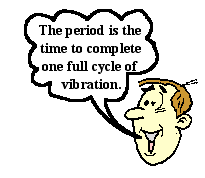An object that is in periodic motion - such as a mass on a spring, a pendulum or a bobblehead doll - will undergo back and forth vibrations about a fixed position in a regular and repeating fashion. The fact that the periodic motion is regular and repeating means that it can be mathematically described by a quantity known as the period. The period of the object's motion is defined as the time for the object to complete one full cycle. Being a time, the period is measured in units such as seconds, milliseconds, days or even years. The standard metric unit for period is the second.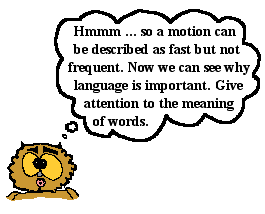An object in periodic motion can have a long period or a short period. For instance, a pendulum bob tied to a 1-meter length string has a period of about 2.0 seconds. For comparison sake, consider the vibrations of a piano string that plays the middle C note (the C note of the fourth octave). Its period is approximately 0.0038 seconds (3.8 milliseconds). When comparing these two vibrating objects - the 1.0-meter length pendulum and the piano string which plays the middle C note - we would describe the piano string as vibrating relatively frequently and we would describe the pendulum as vibrating relatively infrequently. Observe that the description of the two objects uses the terms frequently and infrequently. The terms fast and slow are not used since physics types reserve the words fast and slow to refer to an object's speed. Here in this description we are referring to the frequency, not the speed. An object can be in periodic motion and have a low frequency and a high speed. As an example, consider the periodic motion of the moon in orbit about the earth. The moon moves very fast; its orbit is highly infrequent. It moves through space with a speed of about 1000 m/s - that's fast. Yet it makes a complete cycle about the earth once every 27.3 days (a period of about 2.4x105 seconds) - that's infrequent.

Objects like the piano string that have a relatively short period (i.e., a low value for period) are said to have a high frequency. Frequency is another quantity that can be used to quantitatively describe the motion of an object is periodic motion. The frequency is defined as the number of complete cycles occurring per period of time. Since the standard metric unit of time is the second, frequency has units of cycles/second. The unit cycles/second is equivalent to the unit Hertz (abbreviated Hz). The unit Hertz is used in honor of Heinrich Rudolf Hertz, a 19th century physicist who expanded our understanding of the electromagnetic theory of light waves.

The concept and quantity frequency is best understood if you attach it to the everyday English meaning of the word. Frequency is a word we often use to describe how often something occurs. You might say that you frequently check your email or you frequently talk to a friend or you frequently wash your hands when working with chemicals. Used in this context, you mean that you do these activities often. To say that you frequently check your email means that you do it several times a day - you do it often. In physics, frequency is used with the same meaning - it indicates how often a repeated event occurs. High frequency events that are periodic occur often, with little time in between each occurrence - like the back and forth vibrations of the tines of a tuning fork. The vibrations are so frequent that they can't be seen with the naked eye. A 256-Hz tuning fork has tines that make 256 complete back and forth vibrations each second. At this frequency, it only takes the tines about 0.00391 seconds to complete one cycle. A 512-Hz tuning fork has an even higher frequency. Its vibrations occur more frequently; the time for a full cycle to be completed is 0.00195 seconds. In comparing these two tuning forks, it is obvious that the tuning fork with the highest frequency has the lowest period. The two quantities frequency and period are inversely related to each other. In fact, they are mathematical reciprocals of each other. The frequency is the reciprocal of the period and the period is the reciprocal of the frequency.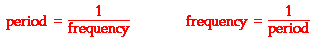This reciprocal relationship is easy to understand. After all, the two quantities are conceptual reciprocals (a phrase I made up). Consider their definitions as restated below:

• period = the time for one full cycle to complete itself; i.e., seconds/cycle
• frequency = the number of cycles that are completed per time; i.e., cycles/second

Even the definitions have a reciprocal ring to them. To understand the distinction between period and frequency, consider the following statement:

According to Wikipedia (and as of this writing), Tim Ahlstrom of Oconomowoc, WI holds the record for hand clapping. He is reported to have clapped his hands 793 times in 60.0 seconds.
What is the frequency and what is the period of Mr. Ahlstrom's hand clapping during this 60.0-second period?

### Amplitude of Vibration

The final measurable quantity that describes a vibrating object is the amplitude. The amplitude is defined as the maximum displacement of an object from its resting position. The resting position is that position assumed by the object when not vibrating. Once vibrating, the object oscillates about this fixed position. If the object is a mass on a spring (such as the discussion earlier on this page), then it might be displaced a maximum distance of 35 cm below the resting position and 35 cm above the resting position. In this case, the amplitude of motion is 35 cm.

Over the course of time, the amplitude of a vibrating object tends to become less and less. The amplitude of motion is a reflection of the quantity of energy possessed by the vibrating object. An object vibrating with a relatively large amplitude has a relatively large amount of energy. Over time, some of this energy is lost due to damping. As the energy is lost, the amplitude decreases. If given enough time, the amplitude decreases to 0 as the object finally stops vibrating. At this point in time, it has lost all its energy.

### We Would Like to Suggest ...Why just read about it and when you could be interacting with it? Interact - that's exactly what you do when you use one of The Physics Classroom's Interactives. We would like to suggest that you combine the reading of this page with the use of our Mass on a Spring Interactive. You can find it in the Physics Interactives section of our website. The Mass on a Spring Interactive provides the learner with a simple environment for exploring the properties of periodic motion.

1. A pendulum is observed to complete 23 full cycles in 58 seconds. Determine the period and the frequency of the pendulum.

2. A mass is tied to a spring and begins vibrating periodically. The distance between its highest and its lowest position is 38 cm. What is the amplitude of the vibrations?

Next Section: